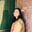Related Tags

diophantine
linear
equation
homogenous
python3

# What is a Diophantine equation?Umme Ammara

A diophantine equation is a polynomial equation that contains two or more unknowns. Only integer solutions are acceptable by the diophantine equation. An integer solution means that all the unknown variables should take integer values.

Diophantine equations can be categorized as follows:

## Linear Diophantine equation

It is the simplest form of the equation, and it follows this format:

ax + by = c.

Here, x and y are unknown variables, and the equation has an integer solution if and only if c is a multiple of the greatest common divisor (GCD) of a and b.

## Homogenous Diophantine equation

A homogenous polynomial defines it, and it follows this format:

$x^{{a}}$ + $y^{{a}}$ - $z^{{a}}$ = 0.

Typically, this equation is the equation of Fermat’s Last Theorem. Solving a homogenous Diophantine equation is generally challenging, as no one has devised a known algorithm that works on all cubic equations. However, there are strategies to solve equations of degree two.

Fermat’s Last Theorem proposes that the Diophantine equation of the format: $x^{{a}}$ + $y^{{a}}$ = $z^{{a}}$, does not have non-zero solutions for a > 2.

## Example

The example below shows how to find the initial integral solution of a linear Diophantine equation in Python. The equation follows the format:

ax + by = c.

We find the values of the unknowns x and y in the following steps:

1. Before moving on to the solution, a few checks are necessary. If a and b are both 0 and c is not 0, there are no solutions to the equation. If c is also 0, then infinite solutions exist.

2. We calculate the Greatest common divisor of a and b by calling the GCD function. This function uses Extended Euclidean Algorithm. Along with the GCD, the function also returns the integers x1 and x2 values.

3. The integral solution of a linear Diophantine equation exists only if the GCD of a and b divides c. If that is the case, we move on to finding the values of x and y.

4. Lastly, we calculate the values of x and y as shown in the code below:

#Function for Extended Euclidean
def GCD(a, b):
if a == 0:
return b, 0, 1
gcd, s, t = GCD(b%a, a)
y1 = s
x1 = t - (b//a) * s
return gcd, x1, y1

#Solve: 3x + 6y = 9
a = 2
b = 5
c = 1

#Step 1
if a==0 and b==0:
if c == 0:
print("Infinite Solutions are possible")
else:
print("Solution not possible")

#Step 2
gcd, x1, y1 = GCD(a,b)

#Step 3 and 4
if (c % gcd == 0):
x = x1 * (c/gcd)
y = y1 * (c/gcd)
print("The values of x and y are: ", x, ",", y)

else:
print("Solution not possible")



RELATED TAGS

diophantine
linear
equation
homogenous
python3

CONTRIBUTORUmme Ammara